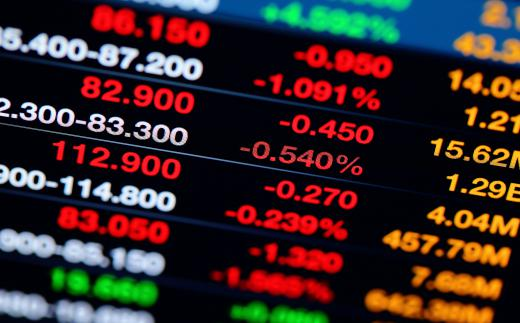Finance
Fact Checked

# What Is a Forward Spread?

K.C. Bruning
K.C. Bruning

A forward spread is the basis point difference between the spot and forward prices of a security. It is specifically the forward price trade discount. The spot price is the current sellable price of a market commodity. A forward price is a pre-determined price agreed upon at the start of a contract that will be paid in the future. The basis point is a unit used to note change in market commodities.

Forward spread is also known as forward pips or forward points. It is commonly used to describe fixed income yields, changes in interest rates, and equity indexes. The usual method of calculating the spread is to compare the forward price with the spot price from the month before. Through this method, it will capture the difference between the two price points in a specific time period.Several different elements are used to calculate the forward spread, including the number of days to delivery of the commodity, the interest rate differential, and the spot price.

Several different elements are used to calculate the forward spread. These include the number of days to delivery of the commodity, the interest rate differential, and the spot price. The result is typically expressed as a single integer and two or more decimal points. For example if a forward price is 1.04 and the spot price from a month before is 1.00, then the forward spread is 0.04. This translates to four basis points.

In some situations, the forward and spot prices are the same. It is then called the at par forward spread. The definition of par is level or equal.A forward spread is the basis point difference between the spot and forward prices of a security.

Some investors may protect themselves from changes in the spread by signing a forward spread agreement. It can be used to lock in a range of rates between which an acceptable basis can be found. In other cases it could involve determining an indexed rate and then agreeing upon how much higher and lower it can be made due to the spread.

In some cases a calculation may be made using multiple forward spreads. This type of forecast usually consists of two separate points ahead of the spot price. While a typical forward spread will capture value at one particular time, two points provide a more detailed picture of the potential future value of a commodity.

A forward spread is one of the simplest ways to forecast market performance. It tends to be a low-risk strategy that can be used easily by investors who are new to trading. This is primarily because the concept is fairly straightforward and does not require solving overly complex equations.

## You might also Like